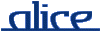# alice library manual.# The MONO_ARRAY signature

## ________ Synopsis ____________________________________________________

```    signature MONO_ARRAY
structure CharArray : MONO_ARRAY where type elem = char
structure Word8Array : MONO_ARRAY where type elem = Word8.word
```

An extended version of the Standard ML Basis' MONO_ARRAY signature.

## ________ Import ______________________________________________________

Imported implicitly.

## ________ Interface ___________________________________________________

```    signature MONO_ARRAY =
sig
type elem
type vector
eqtype array
type t = array

val maxLen :      int

val array :       int * elem -> array
val vector :      array -> vector
val fromList :    elem list -> array
val toList :      array -> elem list
val fromVector :  vector -> array
val toVector :    array -> vector
val tabulate :    int * (int -> elem) -> array

val length :      array -> int
val sub :         array * int -> elem
val update :      array * int * elem -> unit
val swap :        array * int * int -> unit
val rev :         array -> unit
val copy :        {src : array,  dst : array, di : int} -> unit
val copyVec :     {src : vector, dst : array, di : int} -> unit

val app :         (elem -> unit) -> array -> unit
val appr :        (elem -> unit) -> array -> unit
val modify :      (elem -> elem) -> array -> unit
val foldl :       (elem * 'a -> 'a) -> 'a -> array -> 'a
val foldr :       (elem * 'a -> 'a) -> 'a -> array -> 'a
val all :         (elem -> bool) -> array -> bool
val exists :      (elem -> bool) -> array -> bool
val find :        (elem -> bool) -> array -> elem option

val appi :        (int * elem -> unit) -> array -> unit
val appri :       (int * elem -> unit) -> array -> unit
val modifyi :     (int * elem -> elem) -> array -> unit
val foldli :      (int * elem * 'a -> 'a) -> 'a -> array -> 'a
val foldri :      (int * elem * 'a -> 'a) -> 'a -> array -> 'a
val alli :        (int * elem -> bool) -> array -> bool
val existsi :     (int * elem -> bool) -> array -> bool
val findi :       (int * elem -> bool) -> array -> (int * elem) option

val contains :    (elem * elem -> bool) -> array -> elem -> bool
val notContains : (elem * elem -> bool) -> array -> elem -> bool

val equal :       (elem * elem -> bool) -> array * array -> bool
val collate :     (elem * elem -> order) -> array * array -> order

val isSorted :    (elem * elem -> order) -> array -> bool
val sort :        (elem * elem -> order) -> array -> unit
end
```

## ________ Description _________________________________________________

Items not described here are as in the Standard ML Basis' MONO_ARRAY signature.

Note that, unlike polymorphic arrays, monomorphic arrays are strict in their elements. I.e., all constructor and update functions taking individual element values (array, fromList, tabulate, update, modify, modifyi) will request any potential futures immediately.

type t = array

A local synonym for type array.

fromVector v

Creates a vector containing the same elements as the array arr. If v contains more than maxLen elements, then the Size exception is raised.

toVector arr

Creates a vector containing the same elements as the array arr. If arr contains more than Vector.maxLen elements, then the Size exception is raised. Equivalent to vector arr.

toList arr

Creates a list of the elements of arr in order of increasing indices.

rev arr

Reverses in-place the order of elements in array arr.

swap (arr, i, j)

Swaps the ith and jth element of array arr. If i < 0 or |arr| <= i, or j < 0 or |arr| <= j, then the Subscript exception is raised.

appr f arr
appri f arr

Like appi and app, but apply f in right to left order (i.e., decreasing indices). The expression app f arr is equivalent to:

`        appri (f o #2) (arr, 0, NONE)`
alli f arr
existsi f arr

Indexed versions of the functions all and exists. The index of each element is passed to f as an additional argument. The following equivalences hold:

```        all f arr    = alli (f o #2) arr
exists f arr = existsi (f o #2) arr```
contains eq arr a

Returns true if the element a occurs in the array arr, with respect to the element equality eq; otherwise false.

notContains eq arr a

Returns true if the element a does not occur in the array arr, with respect to the element equality eq; otherwise false. Equivalent to not(contains eq arr a).

equal eq (arr1, arr2)

Creates an equality function on arrays given an equality on the element type.

isSorted f arr

Returns true iff arr is sorted with respect to the ordering function f.

sort f arr

Sorts arr with respect to the ordering function f. Sorting may be unstable with respect to equal elements.